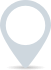# Price elasticity of demand calculator

You can use this price elasticity of demand calculator to calculate the price elasticity of demand.£
.00
£
.00

This calculator is intended for illustration purposes only and exact payment terms should be agreed with a lender before taking out a loan.

Price elasticity of demand

-

Type of elasticity

-

## What is price elasticity of demand?

Price elasticity of demand refers to the measure of the sensitivity of the quantity demanded for a product or service to changes in its price. It reflects how responsive consumers are in adjusting their demand when the price of a product or service changes.

## What are the types of price elasticity of demand?

There are three main types of price elasticity:

1. Elastic demand: This occurs when a small change in price leads to a relatively larger change in quantity demanded. In other words, consumers are highly responsive to price changes, and a price increase results in a significant decrease in demand, while a price decrease leads to a substantial increase in demand. The elasticity coefficient in this case is greater than 1.

2. Inelastic demand: In contrast to elastic demand, inelastic demand describes a situation where a change in price has a relatively smaller impact on quantity demanded. Consumers are less responsive to price changes, and demand remains relatively stable even when prices fluctuate. The elasticity coefficient in this case is less than 1.

3. Unitary elastic demand: Unitary elastic demand occurs when a change in price results in an equivalent percentage change in quantity demanded. In this case, the elasticity coefficient is equal to 1. The percentage change in quantity demanded matches the percentage change in price.

### Clever finance tips and the latest news

#### delivered to your inbox, every week